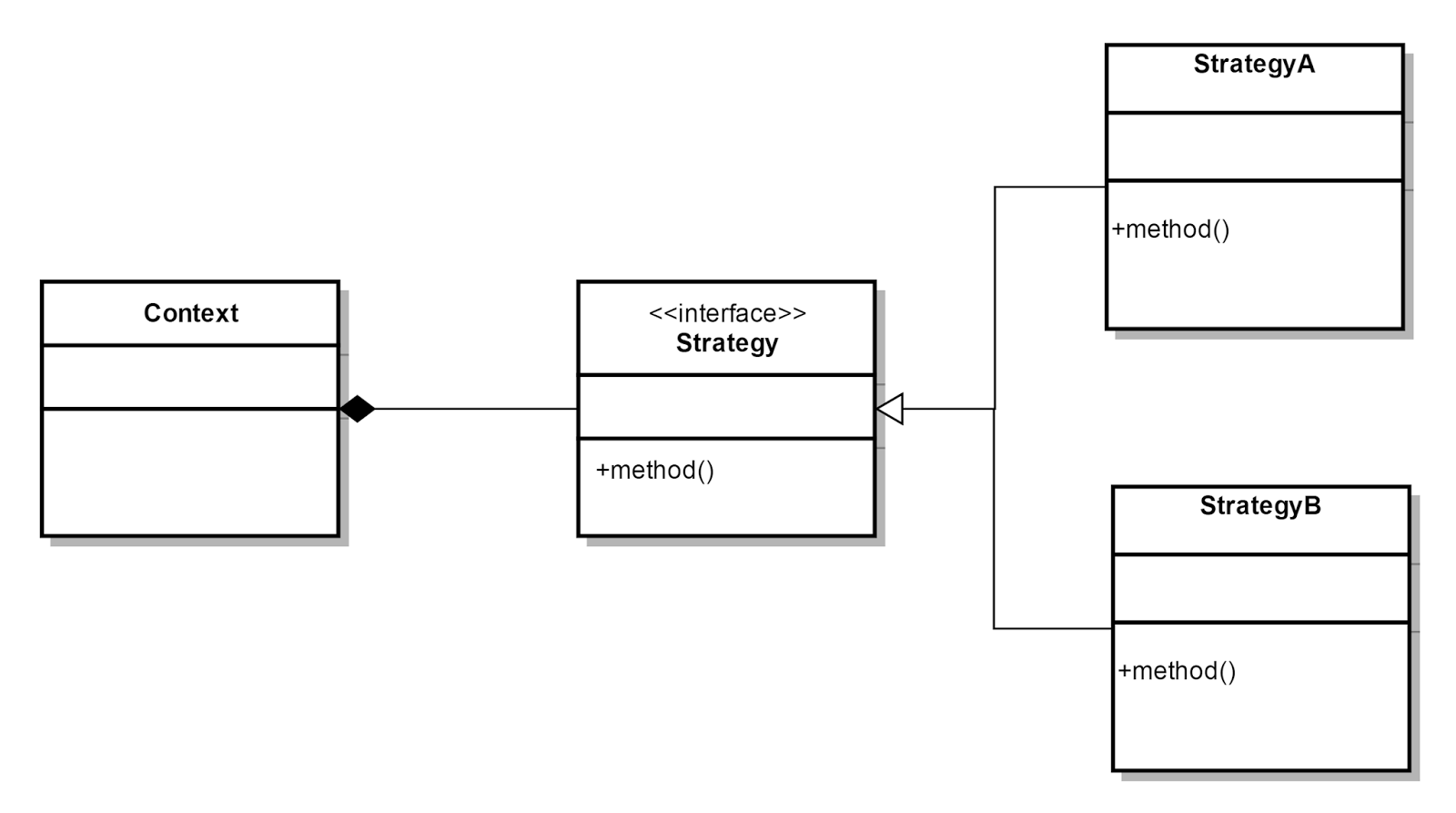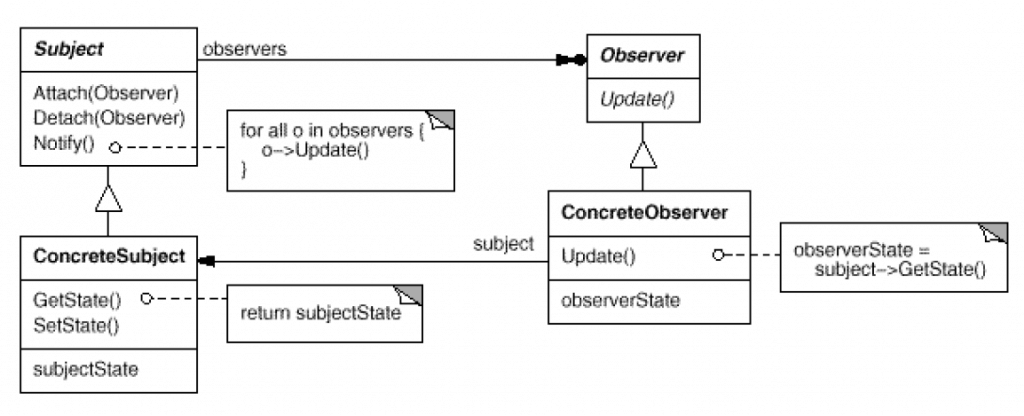X

# “组件协作类”设计模式（C++）

### 模板方法（Template Method）``````class FrameWork {
virtual void startUp() {
std::cout << "[Framework] Initializing framework..." << std::endl;  // default implementaion.
}
virtual void cleanOut() {
std::cout << "[Framework] Cleaning out the resources, and exit..." << std::endl;  // default implementaion.
}
protected:
virtual void core() = 0;  // template function, need to be done by client.
public:
virtual ~FrameWork() {}
void run() {
startUp();
core();
cleanOut();
}
};
class Application : public FrameWork {
void core() override {
std::cout << "[User] Application is running..." << std::endl;
}
};
int main(int argc, char **argv) {
FrameWork *f = new Application();
f->run();
delete f;
return 0;
}
``````

### 策略模式（Strategy）``````struct Strategy {
virtual void strategy() = 0;
virtual ~Strategy() {}
};
class StrategyA : public Strategy {
void strategy() {
std::cout << "[Strategy] StrategyA called..." << std::endl;
}
};
class Application {
std::shared_ptr<Strategy> strategy;
public:
Application(std::shared_ptr<Strategy> strategy) : strategy(strategy) {}
void run() {
std::cout << "[User] Application is running..." << std::endl;
strategy->strategy();
}
};
int main(int argc, char **argv) {
auto strategy = std::make_shared<StrategyA>();  // 可以使用工厂模式进行改进；
auto app = new Application(strategy);
app->run();
delete app;
return 0;
}
``````

• Strategy 及其子类为组件提供了一系列可重用算法，从而可以使得类型在运行时方便地根据需要在各个算法之间进行切换；
• Strategy 模式提供了用条件判断语句以外的另一种选择，消除条件判断语句，就是在解耦合；
• 如果 Strategy 对象没有实例变量，那么各个上下文可以共享同一个 Strategy 对象，从而节省对象开销；

### 观察者模式（Observer / Event）``````struct Observer {
virtual void update(int value) = 0;
};
class Object {  // Subject + Concrete Subject.
int v;
std::list<Observer*> l;
protected:
void notify(int value) {  // notification.
for (auto observer : l) {
observer->update(value);
}
}
public:
Object(int v) : v(v) {};
void attach(Observer* observer) { l.push_back(observer); }
void detach(Observer* observer) { l.remove(observer); }
void increase(int num) {
v+= num;
notify(v);
}
};
struct ObserverA : public Observer {  // Concrete ObserverA.
void update(int value) {
std::cout << "[ObserverA] notified with value: " << value << std::endl;
}
};
class ObserverB : public Observer {  // Concrete ObserverB.
const Object& o;
public:
ObserverB(Object& o) : o(o) { o.attach(this); }  // 组合 Object 到 Observer；
void update(int value) {
std::cout << "[ObserverB] notified with value: " << value << std::endl;
}
};
int main(int argc, char **argv) {
Object o(10);
ObserverA observerA{};
ObserverB observerB{o};
o.attach(&observerA);  // subscription.
// can simulate a timer here.
for (auto i = 0; i < 10; ++i) {
o->increase(100);
}
}, &o).join();
return 0;
}
``````

• 使用面向对象的抽象，Observer 模式使得我们可以独立地改变目标与观察者，从而使二者之间的依赖关系达到松耦合；
• 目标发送通知时，无需指定观察者，通知会自动传播；
• 观察者自己决定是否需要订阅通知，目标对象对此一无所知；
• Observer 模式是基于事件的 UI 框架中非常常用的设计模式，也是 MVC 模式的一个重要组成部分；